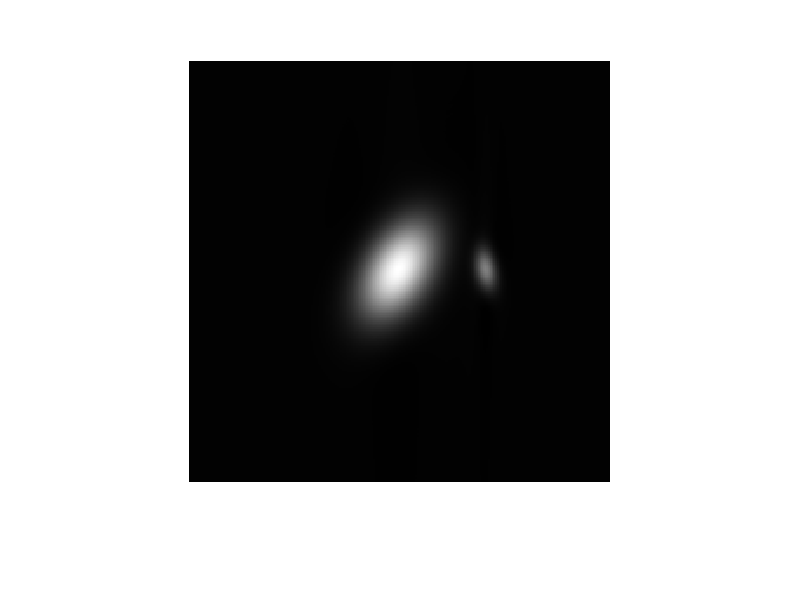If you have trouble viewing this, try the pdf of this post. You can download the code used to produce the figures in this post.

CT Projection Simulator-2

I added a 2D Gaussian object to the projection simulator described in my previous post. I also cleaned up the code.
The Gaussian projections are computed from a formula derived in Bracewell’s book Two Dimensional Imaging (see p. 532). This is a great book and I highly recommend it. To derive the Radon transform of a general Gaussian, Bracewell starts with a circularly symmetric Gaussian. Using his notation where is the Radon transform operator and R is the displacement of the line from the origin
(1) [e − πr2] = e − πR2
He writes e − πr2 = e − π(x2 + y2) and then applies a general coordinate transformation theorem with u = αx and v = βy getting
[e − π(α2x2 + β2y2)] = (exp[ − πR2α2β2 ⁄ (β2cos2(θ) + α2sin2(θ))])/((β2cos2(θ) + α2sin2(θ))).
I think using scaling variables that specify the width of the peak is more intuitive so I substituted a = 1 ⁄ α and b = 1 ⁄ β resulting in
[e − π(x2 ⁄ a2 + y2 ⁄ b2)] = ab(e − πR2 ⁄ sm(θ)2)/(sm(θ))
where sm(θ)2 = a2cos2(θ) + b2sin2(θ).
This formula is similar to the formula for the chord length of an ellipse from my previous post so I could re-use much of the code. The result is included in the code for this post. the code includes an example that creates the projections for two Gaussian blobs and reconstructs it. The results are shown in Fig. 1↓Figure 1 Reconstructed image of two Gaussian blobs created with CTProjSim.
Bracewell’s derivation reminds me of an interesting subset of reconstruction algorithms based on the circular harmonic transform. Eq. 1↑ shows that the exponential is its own Radon transform. That is, it is an eigenfunction of the transform. You can quibble that the transform is really different from the original function--it has different physical units for example--but nevertheless it has the same functional form. Ein-Gal developed a family of other “eigenfunctions” that are not circularly symmetric. Another member, for example is (π)re − πr2eiθ. This is closely related to the Circular Harmonic Transform, which has been widely studied. It was used by Cormack for example in his paper that got him part of the Nobel prize for CT. But as far as I know, it has not resulted in much practical application. The convolution-backprojection algorithm of Bracewell and Riddle is almost universally used. I say almost because there has been a lot of recent interest in iterative algorithms to produce images with reduced noise.
Last edited Sep. 4, 2011
© 2011 by Aprend Technology and Robert E. Alvarez
Linking is allowed but reposting or mirroring is expressly forbidden.

References

 Ronald Newbold Bracewell: Two Dimensional Imaging. Prentice Hall, 1994.

 A. M Cormack: “Representation of a Function by Its Line Integrals, with Some Radiological Applications”, Journal of Applied Physics, pp. 2722—2727, 1963.

 M. Ein-Gal: “The shadow transform: An approach to cross sectional imaging”, NASA STI/Recon Technical Report N, pp. 22706, 1974.

 R. N. Bracewell, A.C. Riddle: “”, The Astrophysical Journal, pp. 427—434, 1967. URL http://ecee.colorado.edu/~ecen4532/spring2010/labs/bracewell.pdf.